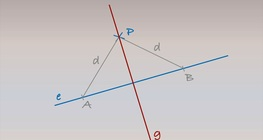Quantity: 0

Total: 0,00

# Constructing the perpendicular bisector of a line segment

### Constructing the perpendicular bisector of a line segment

Let’s construct the perpendicular bisector of a 6-cm-long line segment.

01:36

Mathematics

Keywords

geometry, section, editing, perpendicular, perpendicular to the midpoint, perpendicular bisector, mathematics

Related items

### Related items#### Plotting a perpendicular on a line from a point P that is not on the line

Both line e and point P, which is not situated on line e, are given. Let’s plot line g such that it passes through point P and is perpendicular to line e.#### Plotting a perpendicular to a line at a given point P on the line

Both line e and point P, which is situated on it, are given. Let’s plot line g to include point P on line e so that it is perpendicular to line e.